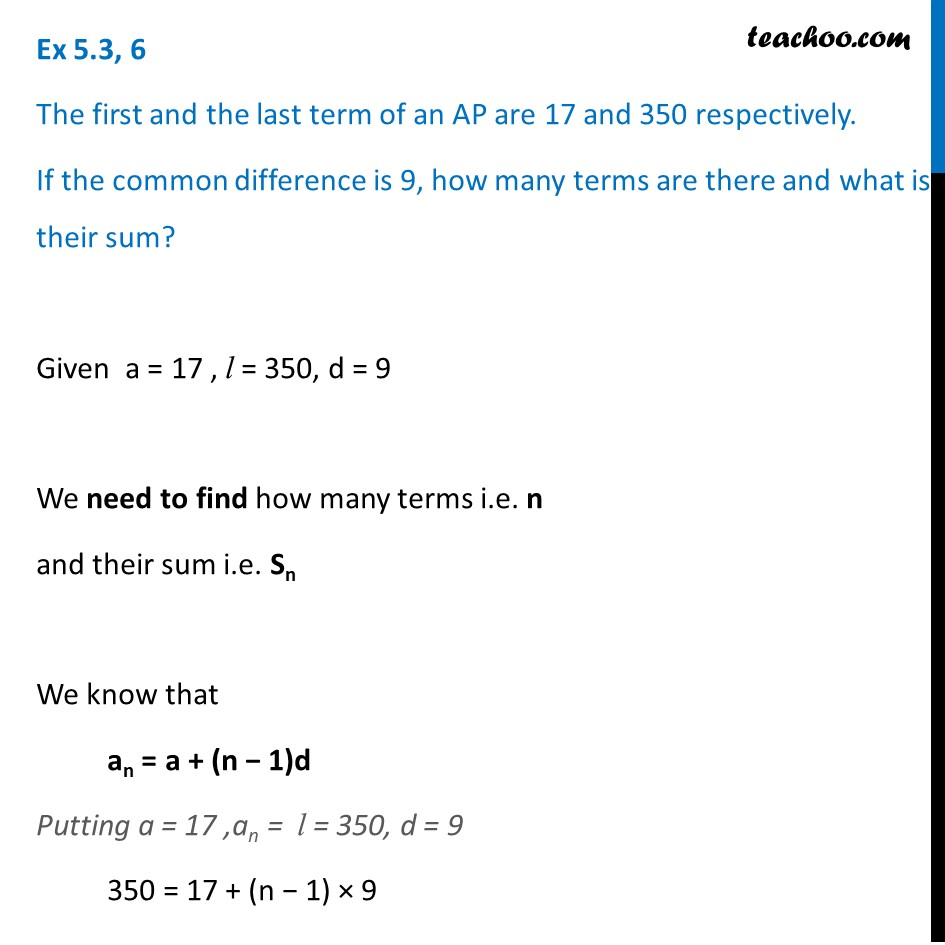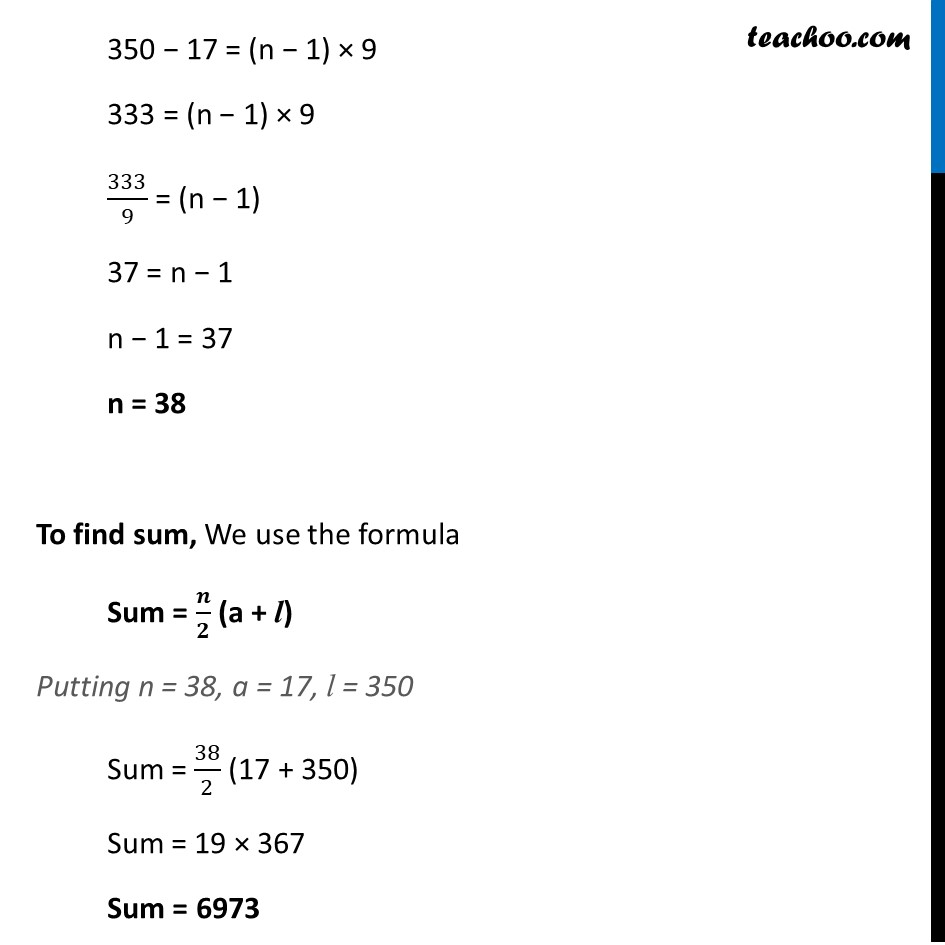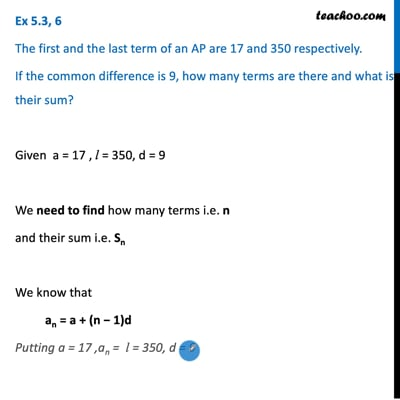Ex 5.3

Chapter 5 Class 10 Arithmetic Progressions
Serial order wiseThis video is only available for Teachoo black users

Solve all your doubts with Teachoo Black (new monthly pack available now!)

### Transcript

Ex 5.3, 6 The first and the last term of an AP are 17 and 350 respectively. If the common difference is 9, how many terms are there and what is their sum? Given a = 17 , l = 350, d = 9 We need to find how many terms i.e. n and their sum i.e. Sn We know that an = a + (n − 1)d Putting a = 17 ,an = l = 350, d = 9 350 = 17 + (n − 1) × 9 350 − 17 = (n − 1) × 9 333 = (n − 1) × 9 333/9 = (n − 1) 37 = n − 1 n − 1 = 37 n = 38 To find sum, We use the formula Sum = 𝒏/𝟐 (a + l) Putting n = 38, a = 17, l = 350 Sum = 38/2 (17 + 350) Sum = 19 × 367 Sum = 6973